# Excel nested IF statement - multiple conditions in a single formula

The tutorial explains how to use multiple IF in Excel and provides a couple of nested If formula examples for most common tasks.

If someone asks you what Excel function you use most often, what would your answer be? In most cases, it's the Excel IF function. A regular If formula that tests a single condition is very straightforward and easy to write. But what if your data requires more elaborate logical tests with multiple conditions? In this case, you can include several IF functions in one formula, and these multiple If statements are called Excel Nested IF. The biggest advantage of the nested If statement is that it allows you to check more than one condition and return different values depending on the results of those checks, all in a single formula.

Microsoft Excel has limits to the levels of nested IFs. In Excel 2003 and lower, up to 7 levels were allowed. In Excel 2007 and higher, you can nest up to 64 IF functions in one formula.

Further on in this tutorial, you will find a couple of Excel nested If examples along with a detailed explanation of their syntax and logic.

## Example 1. Classic nested IF formula

Here's a typical example of Excel If with multiple conditions. Supposing you have a list of students in column A and their exam scores in column B, and you want to classify the scores with the following conditions:

• Excellent: Over 249
• Good: between 249 and 200, inclusive
• Satisfactory: between 199 and 150, inclusive
• Poor: Under 150

And now, let's write a nested IF function based on the above criteria. It's considered a good practice to begin with the most important condition and keep your functions as simple as possible. Our Excel nested IF formula goes as follows:

`=IF(B2>249, "Excellent", IF(B2>=200, "Good", IF(B2>150, "Satisfactory", "Poor")))`

And works exactly as it should: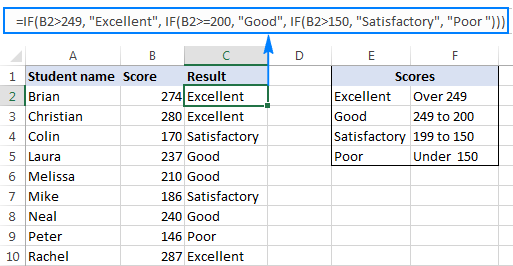### Understanding Excel nested IF logic

I've heard some people say that Excel multiple If is driving them crazy :) Try looking at it at a different angle: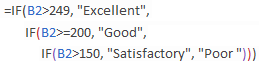What the formula actually tells Excel to do is to evaluate the logical_test of the first IF function and, if the condition is met, return the value supplied in the value_if_true argument. If the condition of the 1st If function is not met, then test the 2nd If statement, and so on.

IF(check if B2>=249, if true - return "Excellent", or else
IF(check if B2>=200, if true - return "Good", or else
IF(check if B2>150, if true - return "Satisfactory", if false -
return
"Poor")))

## Example 2. Multiple If with arithmetic calculations

Here's another typical task: the unit price varies depending on the specified quantity, and your goal is to write a formula that calculates the total price for any amount of items input in a specific cell. In other words, your formula needs to check multiple conditions and perform different calculations depending on what amount range the specified quantity falls in:

 Unit Quantity Price per unit 1 to 10 \$20 11 to 19 \$18 20 to 49 \$16 50 to 100 \$13 Over 101 \$12

This task can also be accomplished by using multiple IF functions. The logic is the same as in the above example, the only difference is that you multiply the specified quantity by the value returned by nested IFs (i.e. the corresponding price per unit).

Assuming the user enters the quantity in cell B8, the formula is as follows:

`=B8*IF(B8>=101, 12, IF(B8>=50, 13, IF(B8>=20, 16, IF( B8>=11, 18, IF(B8>=1, 20, "")))))`

And the result will look something similar to this: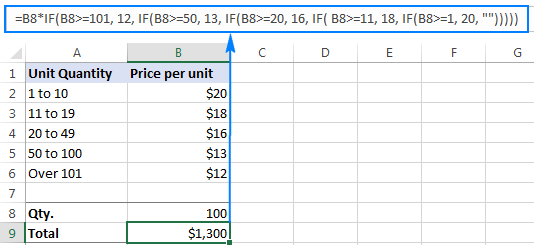As you understand, this example demonstrates only the general approach, and you can easily customize this nested If function depending on your particular task.

For example, instead of "hard-coding" the prices in the formula, you can reference the cells containing those values (cells B2 to B6). This will enable your users to edit the source data without having to update the formula:

`=B8*IF(B8>=101,B6, IF(B8>=50, B5, IF(B8>=20, B4, IF( B8>=11, B3, IF(B8>=1, B2, "")))))`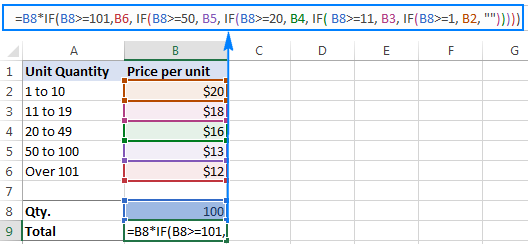Or, you may want to include an additional IF function(s) that fixes an upper, lower or both bounds of the amount range. When the quantity is outside the range, the formula will display an "out of the range" message. For example:

`=IF(OR(B8>200,B8<1), "Qty. out of range", B8*IF(B8>=101,12, IF(B8>=50, 13, IF(B8>=20, 16, IF( B8>=11, 18, IF(B8>=1, 20, ""))))))`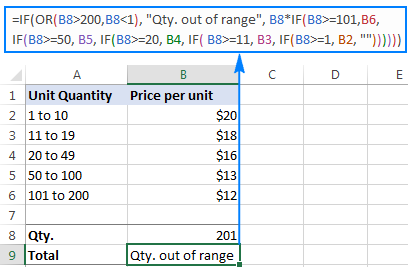The nested IF formulas described above work in all versions of Excel 2016 -2000. In Excel 2016 that is part of Office 365 subscriptions, you can use the IFS function for the same purpose.

Advanced Excel users that are familiar with array formulas, can use this formula that basically does the same thing as the nested IF function discussed above. Though the array formula is far more difficult to comprehend, let along to write, it has one indisputable advantage - you specify the range of cells containing your conditions rather than referencing each condition individually. This makes the formula more flexible, and if your users happen to change any of the existing conditions or add a new one, you will only have to update a single range reference in the formula.

## Excel nested IF - tips and tricks

As you have just seen, there is no rocket science in using multiple IF in Excel. The following tips will help you improve your nested IF formulas and prevent common mistakes.

### Nested IF limits

In Excel 2007 - Excel 365, you can nest up to 64 IF functions. In older versions of Excel 2003 and lower, up to 7 nested IF functions can be used. However, the fact that you can nest a lot of IFs in one formula doesn't mean you should. Please keep in mind that each additional level makes your formula more difficult to understand and troubleshoot. If your formula has too many nested levels, you may want to optimize it by using one of these alternatives.

### The order of nested IF functions matters

The Excel nested IF function evaluates the logical tests in the order they appear in the formula, and as soon as one of the conditions evaluates to TRUE, the subsequent conditions are not tested. In other words, the formula stops after the first TRUE result.

Let's see how it works in practice. With B2 equal to 274, the nested IF formula below evaluates the first logical test (B2>249), and returns "Excellent" because this logical test is TRUE:

`=IF(B2>249, "Excellent", IF(B2>=200, "Good", IF(B2>150, "Satisfactory", "Poor")))`

Now, let's reverse the order of IF functions:

`=IF(B2>150, "Satisfactory", IF(B2>200, "Good", IF(B2>249, "Excellent", "Poor")))`

The formula tests the first condition, and because 274 is greater than 150, the result of this logical test is also TRUE. Consequently, the formula returns "Satisfactory" without testing other conditions.

You see, changing the order of IF functions changes the result: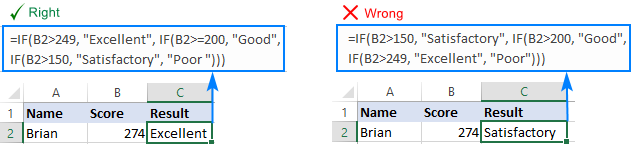### Evaluate the formula logic

To watch the logical flow of your nested IF formula step-by-step, use the Evaluate Formula feature located on the Formula tab, in the Formula Auditing group. The underlined expression is the part currently under evaluation, and clicking the Evaluate button will show you all the steps in the evaluation process.

For example, the evaluation of the first logical test of the nested IF formula shown in the screenshot below will go as follows: B2>249; 274>249; TRUE; Excellent.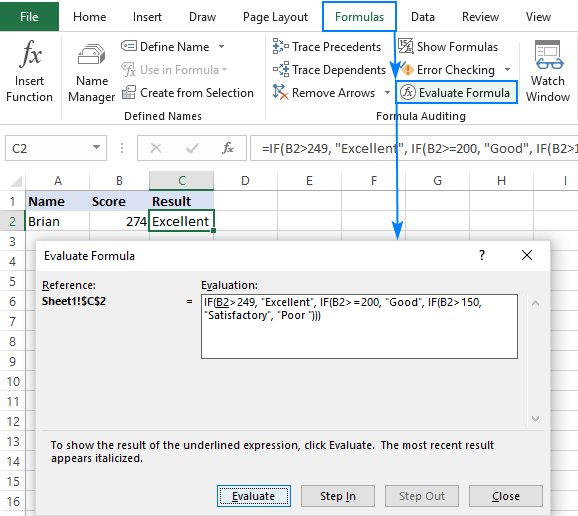### Balance the parenthesis of nested IF functions

One of the main challenges with nested IFs in Excel is matching parenthesis pairs. If the parentheses do not match, your formula won't work. Luckily, Microsoft Excel provides a couple of features that can help you to balance the parentheses when editing a formula:

• If you have more than one set of parentheses, the parenthesis pairs are shaded in different colors so that the opening parenthesis matches the closing one.
• When you close a parenthesis, Excel briefly highlights the matching pair. The same bolding, or "flickering", effect is produced when you move through the formula by using the arrow keys.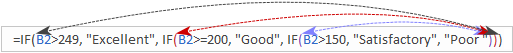### Treat text and numbers differently

When building logical tests of your nested IF formulas, remember that text and numbers should be treated differently - always enclose text values in double quotes, but never put quotes around numbers:

Right: =IF(B2>249, "Excellent",…)

Wrong: =IF(B2>"249", "Excellent",…)

The logical test of the second formula will return FALSE even if the value in B2 is greater than 249. Why? Because 249 is a number and "249" is a numeric string, which are two different things.

### Add spaces or line breaks to make nested IFs easier to read

When building a formula with multiple nested IF levels, you can make the formula's logic clearer by separating different IF functions with spaces or line breaks. Excel doesn't care about extra spacing in a formula, so you may not worry about mangling it.

To move a certain part of the formula to the next line, just click where you want to insert a line break, and press Alt + Enter. Then, expand the formula bar as much as needed and you will see that your nested IF formula has become much easier to understand.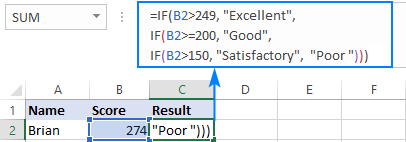## Alternatives to nested IF in Excel

To get around the limit of seven nested IF functions in Excel 2003 and older versions and to make your formulas more compact and fast, consider using the following alternatives to nested Excel IF functions.

1. To test multiple conditions and return different values based on the results of those tests, you can use the CHOOSE function instead of nested IFs.
2. Build a reference table and a use VLOOKUP with approximate match as shown in this example: VLOOKUP instead of nested IF in Excel.
3. Use IF with logical functions OR / AND, as demonstrated in the these examples.
4. Use an array formula like shown in this example.
5. Combine multiple IF statements by using the CONCATENATE function or the concatenate operator (&). A formula example can be found here.
6. For experienced Excel users, the best alternative to using multiple nested IF functions might be creating a custom worksheet function using VBA.

This is how you use an If formula in Excel with multiple conditions. I thank you for reading and hope to see you on our blog next week.

Nested If Excel statements (.xlsx file)

## You may also be interested in

### 485 comments to "Excel nested IF statement - multiple conditions in a single formula"

1.Srinivas says:

Hi,

How to get the formula for the following scenarios

Qty Value Rate Rate 2 Required
18,508 25,96,571 140 - 140
21,716 14,15,208 65 225 65

•Alexander Trifuntov (Ablebits Team) says:

Hi!
What do you want to calculate exactly? Your question is not entirely clear, please specify.

2.Kirk Tindale says:

One number is inputted into column A in either A1, A2 or A3 and then that number is also displayed in column B in either B 1, B2 or B3. But which cell it shows in column B should depend on both the value of the number and the cell in column A it was entered into.

If A1 is lower than 0 then the number is displayed in B3.
If A1 is 0-100 then the number is displayed in B1.
If A1 is over 100 then the number is displayed in B2.

If A2 is lower than 0 then the number is displayed in B1.
If A2 is 0-100 then the number is displayed in B2.
If A2 is over 100 then the number is displayed in B3.

If A3 is lower than 0 then the number is displayed in B2.
If A3 is 0-100 then the number is displayed in B3.
If A3 is over 100 then the number is displayed in B1.

Any idea on how to program this in excel?

•Alexander Trifuntov (Ablebits Team) says:

Hello!
You can use multiple conditions in a nested IF formula:

=IF(AND(A1>0,A1<100),A1,IF(A2<0,A2,IF(A3>100,A3,"")))

This is the formula for cell B1.
I also suggest carefully reading the recommendations in the article above.

3.Ollie says:

Hi I need to calculate the following

If car is 1200cc or lower the and the following will apply and if the car is 1200 of higher the following will apply. Any help would be appreciated

1200cc or lower

up to 1500KM .3795 per KM
1501 to 14000KM .4109 per km
14001 to 25000 .2550 per km

1201cc or higher
up to 1500KM .3986per KM
1501 to 14000KM .4109 per km
14001 to 25000 .2550 per km

Your help would be greatly appreciated on this

Ollie

4.Westberlinbombr says:

Question. I have been racking my brain all night on this.

I have 3 pieces of information that are linked to a master table on another sheet.

I have A1 - Date Assigned
I have A2 - Goal date
I have A3 - Date completed

I want to be able to put in a date into assigned and on my tracker have it read "A" on my master tracker
I am able to calculate a goal date, but want it to show "D" if is goes past the goal date referenced in A2 on the master tracker.
And then I want to be able to put a date in A3 and then have it read "X" for when there is a date listed for completion on my master tracker.

In addition, I want to be able to notice if there are no dates in either A1, A2, or A3 that it just shows blank on the master tracker.

I hope that is enough information to go off of.

•westberlinbombr says:

Also worth noting I have tried to get a formula using =IF(Z6>=TODAY()-(W6*7),AJ6,IF(Z6<=TODAY()-(W6*7),AJ7,IF(AE6,AJ8, "")))

But it will only do the first two it won't allow my third argument to go.

•Alexander Trifuntov (Ablebits Team) says:

Hi!
For example, the formula might look like this:

=IF(Sheet21!A2 < > "",IF(A2>Sheet21!A2,"D",""),"")

5.VJ says:

I have a calculation between 2 cells where, based on the sum, the value should change. If not true, then the value should = the calculated sum. This is the latest attempt but not working. Thanks in advance !

if(H39*0.5)+(H41*0.5)=1.0,"1", if(h39*.05)+(h41*0.5)=2.0,"2", if (h39*.05)+(h41*0.5)=3.0,"3")))

•Alexander Trifuntov (Ablebits Team) says:

Hi!
I fixed the errors in the syntax of the IF function

=IF((H39*0.5)+(H41*0.5)=1,1, IF((H39*0.05)+(H41*0.5)=2,2, IF((H39*0.05)+(H41*0.5)=3,3)))

•VJ says:

Hello, and thank you so much! Getting closer but not quite there.

The problem I have is the results field is formatted with a decimal. When the result is a whole number I do not want the .0 . I have solved for this by using "". However, if the result is not a whole number (1,2, 3.) then I need the calculation to show the results "as is" ( e.g. 0.5). Here is my latest attempt but still not working. Wondering if is should use "or"?... Thanks again!

=IF(H39*0.5+H41*0.5=1,"1",H39*0.5+H41*0.5),IF(H39*0.5+H41*0.5=2,"2",H39*0.5+H41*0.5),IF(H39*0.5+H41*0.5=3,"3",H39*0.5+H41*0.5)

•Alexander Trifuntov (Ablebits Team) says:

Hi!
Change the number format to "General" and you won't see 0 in integers.

=H39*0.5+H41*0.5

Or use the text "1" instead of the number 1.

=IF((H39*0.5)+(H41*0.5)=1,"1",IF((H39*0.05)+(H41*0.5)=2,"2",IF((H39*0.05)+(H41*0.5)=3,"3",H39*0.5+H41*0.5)))

•VJ says:

Hello again and thank you so much for your quick reponse!

Wonderful! I'm going to go with the cell format change to "General". I learned more about the nested IF statement through this exchange but it simplifies the formula, so thank you!

Have a wonderful day!